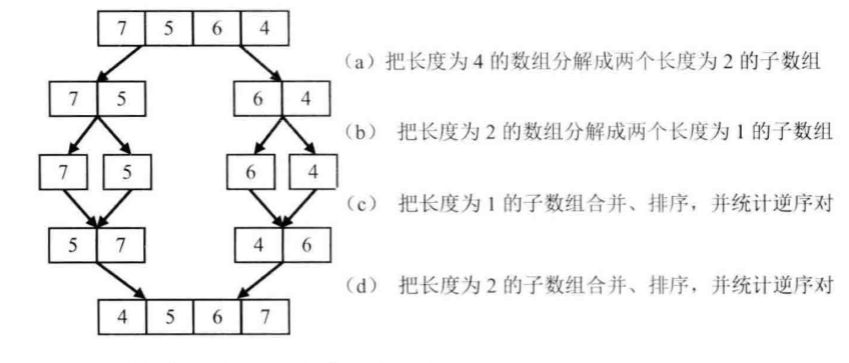# 【剑指Offer】35、数组中的逆序对

题目描述：

在数组中的两个数字，如果前面一个数字大于后面的数字，则这两个数字组成一个逆序对。输入一个数组,求出这个数组中的逆序对的总数P。并将P对1000000007取模的结果输出。 即输出P%1000000007。

输入描述：

题目保证输入的数组中没有的相同的数字数据范围：

• 对于%50的数据,size<=10^4
• 对于%75的数据,size<=10^5
• 对于%100的数据,size<=2*10^5

解题思路：

本题一个最容易想到的解法是暴力解法，顺序扫描整个数组，每扫描到一个数字时，逐个比较该数字与后面的数字的大小关系，统计逆序对的个数，假设数组中有n个数字，则每个数字都要和O(n)个数字做比较，因此，这个暴力解法的时间复杂度为O(n^2)。

一般情况下，最容易想到的往往不是最优解法。在这里，我们采用分治的思想，类比归并排序算法来分析此题。

首先将数组分隔成子数组，统计出子数组内部逆序对数目，然后再统计相邻子数组之间的逆序对数目，统计过程中还需要对数组进行排序，这实际上就是归并排序的过程。主要考虑的是合并两个有序序列时，计算逆序对数。对于两个有序升序序列，设置两个下标分别指向开始位置，每次比较两个指针对应的值，如果第一个序列当前值大于第二个序列当前值，则有第一个序列“当前长度”个逆序对。

这看起来好像比较拗口，但是从代码中可以直观看出。

举例：编程实现（Java）：

public class Solution {
int res=0;
public int InversePairs(int [] array) {
//数组中的逆序对，归并排序
if(array==null || array.length==0)
return 0;
findInversePairs(array,0,array.length-1);
return res%1000000007;
}
public void findInversePairs(int[] array,int low,int high){
if(low<high){
int mid=low+(high-low)/2;
findInversePairs(array,low,mid); //左一半递归
findInversePairs(array,mid+1,high); //右一半递归
//merge
merge(array,low,mid,high);
}
}
public void merge(int[] array,int low,int mid,int high){
int i=low,j=mid+1;
int[] temp=new int[high-low+1];
int k=0;
while(i<=mid && j<=high){
if(array[i]<=array[j])
temp[k++]=array[i++];
else {   //大于，说明是逆序
temp[k++] = array[j++];
res += (mid - i + 1);
res = res%1000000007;
}
}
while(i<=mid)
temp[k++]=array[i++];
while(j<=high)
temp[k++]=array[j++];

for(i=0;i<temp.length;i++)
array[low+i]=temp[i];
}
}

posted @ 2019-05-08 20:02  gzshan  阅读(2000)  评论(0编辑  收藏  举报